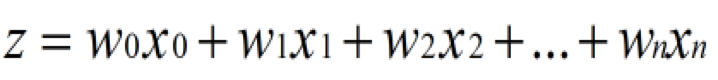# Bob's Blog

Web开发、测试框架、自动化平台、APP开发、机器学习等

### 机器学习(二)之逻辑回归

1）Sigmoid函数

Sigmoid是激活函数中的一种。在我理解来看，逻辑回归和线性回归有比较大的相似处，但由于逻辑回归是需要输出分类，那么在数值的处理上就需要一个转换，sigmoid就在这里产生作用。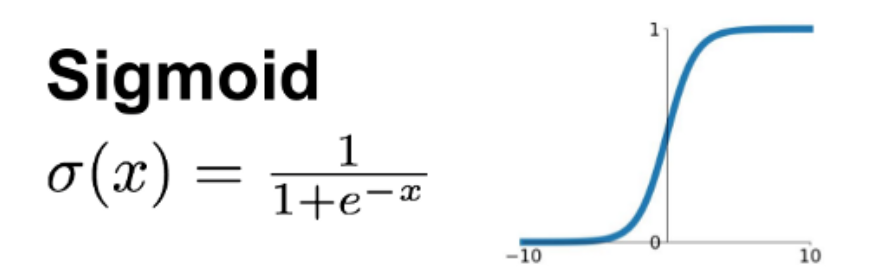2）梯度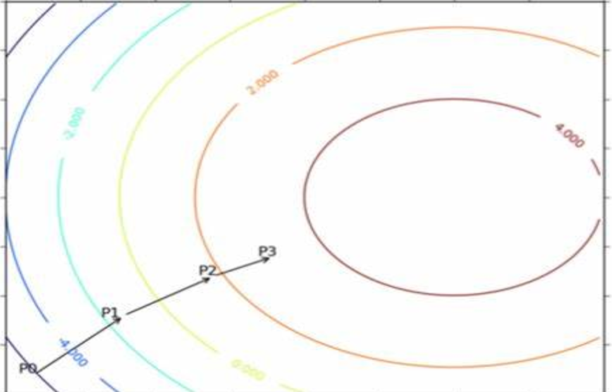3）回归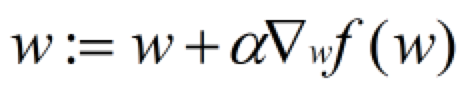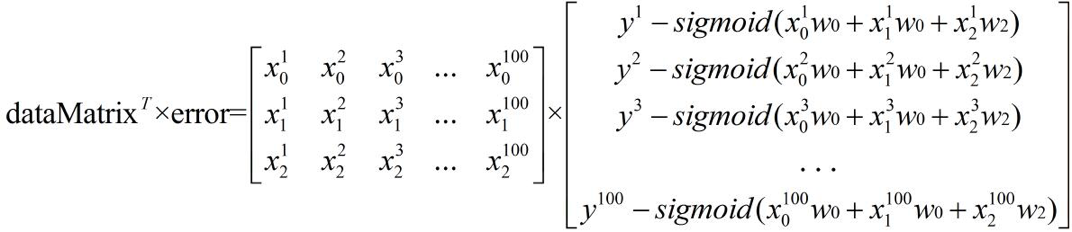``````# set iteration
def asc(data_mat, label_mat, step_size=0.001, max_iter=1000):
data_mat_np = np.mat(data_mat)
label_mat_np = np.mat(label_mat).transpose()
m, n = np.shape(data_mat_np)
weights = np.ones((n, 1))
for _ in range(max_iter):
h = sigmoid(data_mat_np * weights)
error = label_mat_np - h
weights = weights + step_size * data_mat_np.transpose() * error
return weights.getA()``````

``````# set target and timeout
def asc_with_target(data_mat, label_mat, step_size=0.001, target=0.9, timeout=3600):
data_mat_np = np.mat(data_mat)
label_mat_np = np.mat(label_mat).transpose()
m, n = np.shape(data_mat_np)
weights = np.ones((n, 1))
loop_count = 0
total = len(data_mat)
temp_match_rate = 0
interval = 0
start = time.time()
while True:
loop_count += 1
interval += 1
h = sigmoid(data_mat_np * weights)
error = label_mat_np - h
trained_result = []
for i in h:
trained_result.append(classify(i))
compare_result = np.unique(np.array(trained_result) - np.array(label_mat), return_counts=True)
matched_index = compare_result.tolist().index(0)
matched_count = compare_result.tolist()[matched_index]
current_match_rate = matched_count / total
if current_match_rate > target:
if temp_match_rate > current_match_rate:
break
if interval >= timeout:
interval = 0
end = time.time()
if end - start >= timeout:
break
temp_match_rate = current_match_rate
weights = weights + step_size * data_mat_np.transpose() * error
return weights.getA()``````

4）随机抽样

---------------------------------------------------------